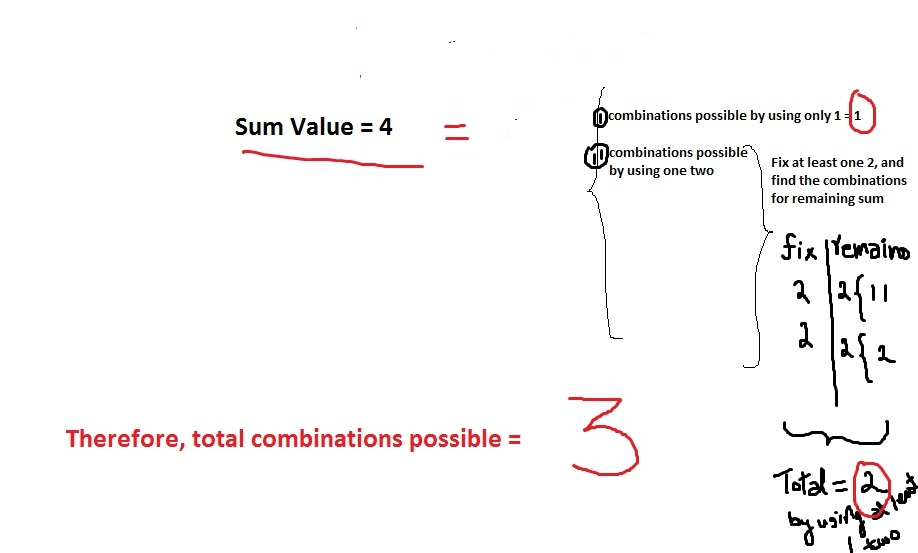# CLEAN CODE: THE COIN CHANGE PROBLEM DYNAMIC PROGRAMMING

By: Saurav

2017-12-28 09:33:00 UTC

You are given a set of coins S. In how many ways can you make sum N assuming you have infinite amount of each coin in the set.

Note : Coins in set S will be unique. Expected space complexity of this problem is O(N).

Example :

Input :
S = [1, 2, 3]
N = 4

Return : 4

Explanation : The 4 possible ways are
{1, 1, 1, 1}
{1, 1, 2}
{2, 2}
{1, 3}

Hacker Rank

This problem is the starting dynamic problem taught in any class. But if we go directly to solving the problem with dynamic programming, it won't serve the purpose.

Normally, you use DP when something is repetitive as per step 4 in my blog post .

When we need to find the total combination/ways of arranging an amount when given coins of a specific denomination is present, you add the combinations possible without taking that coin in combination possible when at least one coin present as indicated in the figure below:

Lets take an example of two types of coins with value 1 and 2. We need to find how many combinations are possible with these.
We start with 1.

We can write a recursive approach as indicated here. That recursive approach should be the way to figure out what's being repeated and what we can memorize and store using DP as shown in the code below:```nm  = gets.split(" ")
n = nm.to_i
m = nm.to_i

coins = gets.split(" ").map{|i| i.to_i}

def get_number_of_ways(n,m,coins)
return 0 if m < 1
coins.sort!

dp_array = Array.new(coins.size+1){Array.new(n+1)}

(0..m).each do |i|
(0..n).each do |j|
dp_array[i][j] = 0 if i == 0
dp_array[i][j] = 1 if j == 0
dp_array[i][j] = 1 if ((j > 0) and (i > 0) and (coins[i-1] == 1))

if ((j > 0) and (i > 0) and coins[i-1] != 1)
if j >= coins[i-1]
dp_array[i][j] = dp_array[i-1][j] + dp_array[i][j-coins[i-1]]
else
dp_array[i][j] = dp_array[i-1][j]
end
end

end
end

return dp_array[-1][-1]

end
puts get_number_of_ways(n,m,coins)```

Looks pretty good to me.

### Let me know what you think! twitter: sprakash24octlinkedin

Owned & Maintained by Saurav Prakash

If you like what you see, you can help me cover server costs or buy me a cup of coffee though donation :)